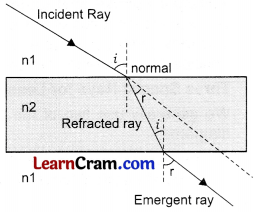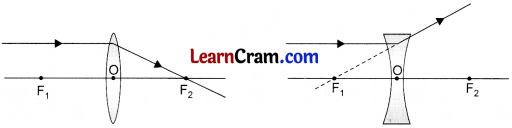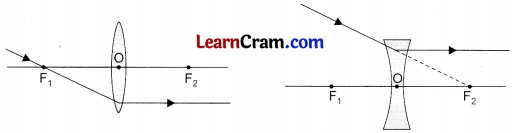# DAV Class 8 Science Chapter 10 Notes – Refraction and Dispersion of Light

These DAV Class 8 Science Notes and DAV Class 8 Science Chapter 10 Notes – Refraction and Dispersion of Light act as excellent revision resources, particularly in preparation for board exams.

## Refraction and Dispersion of Light Class 8 DAV Notes

→ Refraction of Light: When a ray of light travels from one transparent medium to another transparent medium, it changes its direction of propagation. This phenomenon is called refraction of light.

→ Causes of Refraction of Light:

• Change in the speed of light from one medium to another is the cause of refraction of light.
• Optically rarer medium: If the speed of light in a given medium is higher, the medium is called optically rarer medium.
• Optically denser medium: If the speed of light in a given medium is lower, the medium is called optically denser medium.
• Speed of light in vacuum = 3 × 108 m per second

→ Refractive Index: The ratio of speeds of light; in a given pair of media gives the value of relative refractive index for the given pair of media.

Relative refractive index of medium 2 with respect to medium 1
1²μ = $$\frac{\text { Speed of light in medium 1 }}{\text { Speed of light in medium 2 }}=\frac{v_1}{v_2}$$
The refractive index or absolute refractive index of a medium (m), denoted by (μm) is given as follows:
μm = $$\frac{\text { Speed of light in vacuum C }}{\text { Speed of light in the given medium }}=\frac{C}{v_m}$$→ Rules for Refraction:
1. When a ray of light travels (obliquely) from an optically rarer medium to an optically denser medium, it bends towards the normal.2. When a ray of light travels (obliquely) from an optically denser medium to an optically rarer medium, it bends away from the normal.

→ Manifestations of refraction of light:
A drinking straw appears bent from near the surface of water. This happens because of refraction of light.
>• A coin kept in water appears shallower than it actually is. This also happens because of refraction of light.

→ Some Definitions:• Incident Ray: The ray falling on the surface of second medium.
• Refracted Ray: The ray travelling through the second medium.
• Emergent Ray: The ray emerging into the first medium.
• Angle of Incidence: The angle between the incident ray and the normal is called angle of incidence. It is written as ∠i.
• Angle of Refraction: The angle between the refracted ray and the normal is called angle of refraction. It is written as ∠r.
• Lateral Deviation: The apparent displacement in the path of ray of light; once it emerges from the second medium; is called lateral deviation

→ Dispersion of White Light by a Glass Prism: When white light passes through a glass prism, it segregates into its component colours. This phenomenon is called dispersion of light. The speed of light of different colours are different. Different colours deviate differently because of the difference in their speed. This is the reason of dispersion of white light when it passes through a glass prism.→ Rainbow Formation: Rainbow is formed just after the rain. When the sunlight passes through water droplets, it gets dispersed. The water droplet works as a prism in this case. Rainbow is formed due to dispersion of light.

→ Lens: A lens is a portion of a transparent refracting medium which is bound by two surfaces. At least one of the surfaces is spherical in a lens.

→ Types of Spherical Lens:→ Some Definitions:
Centres of Curvature: Each of the two surfaces of a spherical lens can be regarded as a part of a sphere. The centres of these two spheres are known as the centres of curvature.

→ Radii of Curvature: The radii of two spheres; of which the lens surfaces are a part; are known as the radii of curvature.
Principal Axis: The line joining the centres of curvature of the two surfaces is called the principal axis.

→ Optical Centre: The mid-point of the lens is called the optical centre of the lens. A ray of light passing through the optical centre of a lens goes through it without undergoing any deviation. Principal Focus/Focal Length: The mid-point between the optical centre and the centre of curvature is the focus of the lens. If a beam of rays, all parallel to the principal axis, falls on the lens they either converge at or diverge from the principal focus of the lens. The distance of the principal focus from the optical centre is called the focal length of the lens.

→ Three Special Rays for Lenses:
We generally use two of the three (special) incident rays, described below to draw the ray diagrams:
1. An incident ray, parallel to the principal axis, passes through or appears to come from, the second principal focus of the lens.2. An incident ray, passing through the optical centre of the lens, goes undeviated from the lens.3. An incident ray, passing through the first principal focus of the lens, or directed towards it, becomes parallel to the principal axis after refraction from the lens.→ Image formation by concave lens : In case of concave lens, image is virtual and erect. It is smaller than the object and is formed between F and O on the same side of the lens. This happens in all cases; irrespective of the position of the object.→ Applications of Lenses: In camera, microscope, telescope and spectacles.

→ Refraction of Light : The deviation in the path of ray of light when it travels from one medium to another is called refraction of light.

→ Dispersion of light : When white light travels through a glass prism, it segregates into its seven component colours. This phenomenon is called dispersion of light.

→ Lens : A part of transparent refractive medium which is bound by two surfaces and at least one surface ¡s part of a sphere.

→ VIBGYOR : This is an anagram for different colours of the rainbow, i.e. violet. indigo, blue, green, yellow, orange and red.

→ Image formation in convex lens:

 Position of object Detail of image Nature Size Position At infinity Real and inverted Very small At focus (on the other side) Beyond 2F Real and inverted Smaller than the object Between F and 2F (on the other side) At 2F Real and inverted Same as the object At 2F (on the other side) Between F and 2F Real and inverted Larger than the object Beyond 2F (on the other side) At F Real and inverted Very large At infinity (on the other side) Between F and O Virtual and erect Larger than the object Between F and 2F (on the same side)→ Representative Values of Speed of Light in Some Media

 Medium Vacuum Air Water Glass (ordinary) Diamond Speed of light (nearly) in lakhs of km/s 3 ~3 ~2.25 ~2 ~1.25